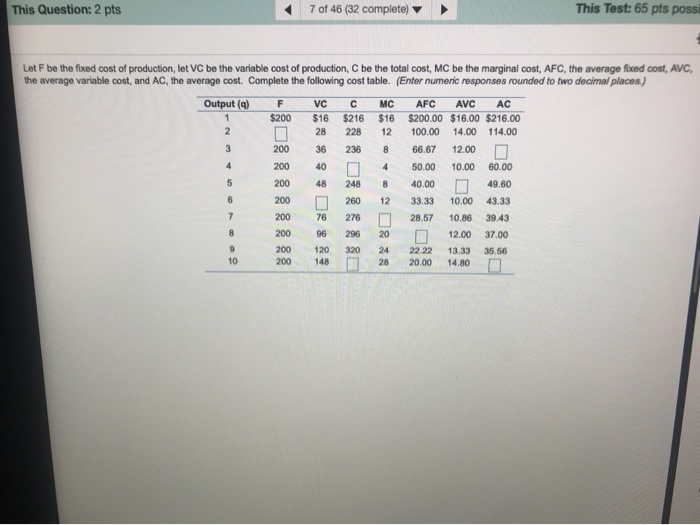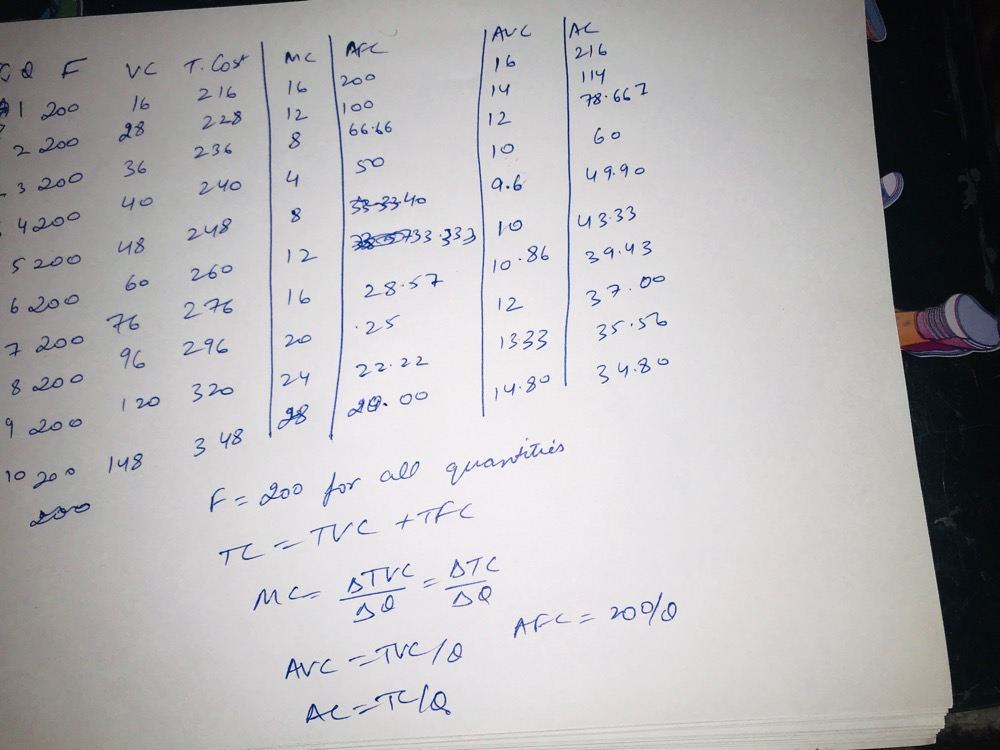Question

# This Question: 2 pts 7 of 46 (32 complete) This Test: 65 pts possi Let F...This Question: 2 pts 7 of 46 (32 complete) This Test: 65 pts possi Let F be the fixed cost of production, let VC be the variable cost of production, C be the total cost. MC be the marginal cost, AFC, the average fixed cost, AVC the average variable cost, and AC, the average cost. Complete the following cost table. (Enter numeric responses rounded to two decimal places) Output (a) F \$200 1 VCC \$16 \$216 28 228 36 236 40 0 248 260 276 296 320 MC AFC AVC AC \$16 \$200.00 \$16.00 \$216.00 12 100.00 14.00 114.00 8 66.67 12.000 4 50.00 10.00 60.00 8 40.000 49.60 12 33.33 10.00 43.33 0 28.57 10.86 39.43 200 12.00 37.00 24 22 22 13.33 35.56 28 20.00 14.80#### Earn Coins

Coins can be redeemed for fabulous gifts.

Similar Homework Help Questions
• ### Let F be the fixed cost of production, let VC be the variable cost of production,...Let F be the fixed cost of production, let VC be the variable cost of production, C be the total cost, MC be the marginal cost, AFC, the average faced cost AVC, the average variable cost, and AC, the average cost. Complete the following cost table. (Entor numeric responses rounded to two decimal places) Output (a) \$264 312 MC AFC AVC AC \$64 \$200.00 \$64.00 \$264.00 48 100.00 56.00 156.00 66.67 48.00 50.00 40.00 90.00 N 78.40 33.33 40.00 73.33...